Courses

# Factorisation of Polynomials and Factor Theorem - Polynomials, Class 9, Mathematics | EduRev Notes

## Class 9 : Factorisation of Polynomials and Factor Theorem - Polynomials, Class 9, Mathematics | EduRev Notes

The document Factorisation of Polynomials and Factor Theorem - Polynomials, Class 9, Mathematics | EduRev Notes is a part of Class 9 category.
All you need of Class 9 at this link: Class 9

FACTOR THEOREM
If p(x) is a polynomial of degree n ≥ 1 and a is any real number, then

(i) x – a is a factor of p(x), then p(a) = 0 and

(ii) p(a) = 0 then x – a is a factor of p(x).

 REMARK (x + a) is a factor of p(x) ⇔ p(–a) = 0  (ax – b) is a factor of p(x) ⇔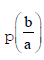= 0  (ax + b) is a factor of p(x) ⇔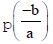= 0 (x – a) (x – b) is a factor of a polynomial p(x) ⇔ p(a) = 0, p(b) = 0

Ex. Use the factor theorem to determine whether x – 1 is a factor of

(a) x3 + 8x2 – 7x – 2

(b) 2√2 x3 + 5√2 x2 – 7√2

Sol. (a) Let p(x) = x3 + 8x2 – 7x – 2

By using factor theorem, (x – 1) is a factor of p(x) only when p(1) = 0

p(1) = (1)3 + 8(1)2 – 7(1) – 2 = 1 + 8 – 7 – 2 = 9 – 9 = 0

Hence (x – 1) is a factor of p(x).

(b) Let p(x) = 2√2x3 + 5√2x2 – 7√2

By using factor theorem, (x – 1) is a factor of p(x) only when p(1) = 0

p(1) = 2√2 (1)3 + 5√2 (1)2 – 7√2 = 2√2 + 5√2 – 7√2 = 7√2 – 7√2 = 0

Hence (x – 1) is a factor of p(x).

Ex. Without actual division, prove that the polynomial 2x3 + 13x2 + x – 70 is exactly divisible by x – 2.

Sol. The polynomial p(x) = 2x3 + 13x2 + x – 70 is exactly divisible by x – 2 means that x – 2 is a factor of

p(x) = 2x3 + 13x2 + x – 70.

Now p(2) =2(2)3 + 13(2)2 + 2 – 70 = 16 + 52 + 2 – 70 = 0

∴ By factor theorem, x – 2 is a factor of p(x)

i.e. p(x) = 2x3 + 13x2 + x – 70 is exactly divisible by x – 2.

FACTORISING OF POLYNOMIAL OF HIGHER DEGREE

1. Obtain the polynomial p(x)
2. Obtain the constant term in p(x) and find its all possible factors. For example, in the polynomial x4 + x3 – 7x2 – x + 6 the constant term is 6 and its factors are ±1, ±2, ±3, ±6.
3. Take one of the factors, say a and replace x by it in the given polynomial. If the polynomial reduces to zero, then (x – a) is a factor of polynomial.
4. Obtain the factors equal in number to the degree of polynomial. Let these are (x – a), (x – b), (x – c). ....
5. Write p(x) = k (x – a) (x – b) (x – c) ..... where k is constant.
6. Substitute any value of x other than a, b, c .......... and find the value of k.

Ex. Using factor theorem, factorize the polynomial x3 – 6x2 + 11x – 6.
Sol. Let f(x) = x3 – 6x2 + 11x – 6

The constant term in f(x) is equal to –6 and factors of – 6 are ±1, ±2, ±3, ±6. Putting x = 1 in f(x), we have

f(1) = (1)3 – 6 × (1)2 + 11 × 1 – 6 = 1 – 6 + 11 – 6 = 0     ∴        (x – 1) is a factor of f(x)

Similarly, x – 2 and x – 3 are factors of f(x). Since f(x) is a polynomial of degree 3. So, it can not have more than three linear factors.

Let f(x) = k (x – 1) (x – 2) (x – 3)

Then,x3 – 6x2 + 11x – 6 = k(x – 1) (x – 2) (x – 3)

Putting x = 0 on both sides, we get – 6 = k (0 – 1) (0 – 2) (0 – 3) ⇒   –6 = – 6 k      ⇒   k = 1

Putting k = 1 in f(x) = k(x – 1) (x – 2) (x – 3), we get f(x) = (x – 1) (x – 2) (x – 3)

Hence, x3 – 6x2 + 11x – 6 = (x – 1) (x – 2) (x – 3)

Ex. Using factor theorem, factorize the polynomial x4 + x3 – 7x2 – x + 6.

Sol. Let f(x) = x4 + x3 – 7x2 – x + 6 the factors of constant term 6 are ±1, ±2, ±3 and ±6

Now, f(1) = 1 + 1 – 7 – 1 + 6 = 8 – 8 = 0

⇒ (x – 1) is a factor of f(x)

f(–1) = 1 – 1 – 7 + 1 + 6 = 8 – 8 = 0     ⇒     (x + 1) is a facor of f(x)

f(2) = 24 + 23 – 7 × 22 – 2 + 6 = 16 + 8 – 28 – 2 + 6 = 0

⇒ x – 2 is a factor of f(x)

f(–2) = (–2)4 + (–2)3 – 7(–2)2 – (–2) + 6 = 16 – 8 – 28 + 2 + 6 = – 12    ≠ 0

⇒ x + 2 is not a factor of f(x)

f(–3) = (–3)4 + (–3)3 – 7(–3)2 – (–3) + 6 = 81 – 27 – 63 + 3 + 6 = 90 – 90 = 0

⇒ x + 3 is a factor of f(x).

Since f(x) is a polynomial of degree 4. So, it cannot have more than 4 linear factors

Thus, the factor of f(x) are (x – 1), (x + 1), (x – 2) and (x + 3).

Let f(x) = k(x – 1) (x + 1) (x – 2) (x + 3)

⇒ x4 + x3 – 7x2 – x + 6 = k (x – 1) (x + 1) (x – 2) (x + 3) ... (i)

Putting k = 0 on both sides, we get

6 = k (–1) (1) (–2) (3)        ⇒     6 = 6 k          ⇒          k = 1

Substituting k = 1 in (i), we get

x4 + x3 – 7x2 – x + 6 = (x – 1) (x + 1) (x – 2) (x + 3)

 COMPETITION WINDOW ZERO OF A QUADRATIC POLYNOMIAL Symmetric function : An algebraic expression in α and β, which remains unchanged, when α and β are interchanged is known as symmetric function in α and β. For example,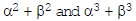etc. are symmetric functions. Symmetric function is to be expressed in terms of (α+ β) and αβ. So, this can be evaluated for a given quadratic equation. Some useful relations involving α and β :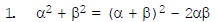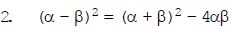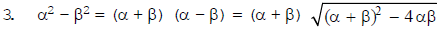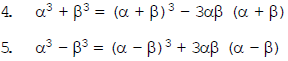Ex. If α and β are the zeroes of the polynomial ax2 + bx + c. Find the value of (i) α – β (ii) α2 + β2 Sol. Since α and β are the zeroes of the polynomial ax2 + bx + c.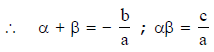(i) (α – β)2 = (α + β)2 – 4αβ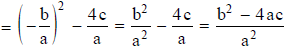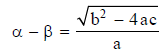(ii) α2 + β2 = α2 + β2 + 2αβ – 2αβ = (α + β)2 – 2αβ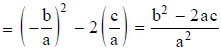Ex. If α and β are the zeroes of the quadratic polynomial ax2 + bx + c. Find the value of (i) α2 – β2 (ii) α3 + β3. Sol. Since α and β are the zeroes of ax2 + bx + c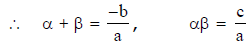(i) α2 – β2 = (α + β) (α – β)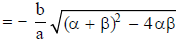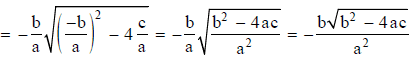(ii) α3 + β3 = (α + β) (α2 + β2 – αβ) = (α + β) [(α2 + β2 + 2αβ) – 3αβ] = (α + β) [(α + β)2 – 3αβ] =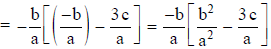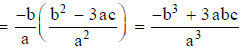TO FORM A QUADRATC POLYNOMIAL WITH THE GIVEN ZEROES Let the zeroes of a quadratic polynomial be α and β. ∴ x = α,               x = β    x – α = 0,          x – β = 0 The obviously the quadratic polynomial is (x – α) (x – β) i.e., x2 – (α + β) x + αβ x2 – (Sum of the zeroes) x + Product of the zeroes Ex. Form the quadratic polynomial whose zeroes are 4 and 6. Sol. Sum of the zeroes = 4 + 6 = 10 Product of the zeroes = 4 × 6 = 24 Hence the polynomial formed = x2 – (sum of zeroes) x + Product of zeroes = x2 – 10x + 24 Ex. Form the quadratic polynomial whose zeroes are –3, 5 Sol. Here, zeroes are – 3 and 5. Sum of the zeroes = –3 + 5 = 2 Product of the zeroes = (–3) × 5 = –15 Hence the polynomial formed = x2 – (sum of zeroes) x + Product of zeroes = x2 – 2x – 15
Offer running on EduRev: Apply code STAYHOME200 to get INR 200 off on our premium plan EduRev Infinity!

,

,

,

,

,

,

,

,

,

,

,

,

,

,

,

,

,

,

,

,

,

,

,

,

,

,

,

;